ConicBundle

base class for collecting and organizing a sequence of changes to linear data so that it can be carried out in one step later on; this class comprises all features, derived ones are specializations with partial reinterpretations. More...

#include <Modification.hxx>

Inheritance diagram for ConicBundle::Modification: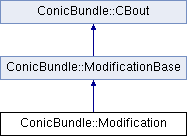Public Member Functions

Constructors and initialization
virtual ~Modification ()

Modification (CH_Matrix_Classes::Integer var_olddim, CH_Matrix_Classes::Integer row_olddim, const CBout *cb=0, int incr=-1, bool ensure_start_val_box_feasibility=false, bool ensure_bounds_consistency=true, CH_Matrix_Classes::Real start_val_def=0., CH_Matrix_Classes::Real bounds_minus_infty=CB_minus_infinity, CH_Matrix_Classes::Real bounds_plus_infty=CB_plus_infinity, CH_Matrix_Classes::Real rhs_minus_infty=CB_minus_infinity, CH_Matrix_Classes::Real rhs_plus_infty=CB_plus_infinity, CH_Matrix_Classes::Real cost_def=0.)
calls clear() with these parameters and initializes CBout

int clear (CH_Matrix_Classes::Integer var_olddim, CH_Matrix_Classes::Integer row_olddim, bool ensure_start_val_box_feasibility=false, bool ensure_bounds_consistency=true, CH_Matrix_Classes::Real start_val_def=0., CH_Matrix_Classes::Real bounds_minus_infty=CB_minus_infinity, CH_Matrix_Classes::Real bounds_plus_infty=CB_plus_infinity, CH_Matrix_Classes::Real rhs_minus_infty=CB_minus_infinity, CH_Matrix_Classes::Real rhs_plus_infty=CB_plus_infinity, CH_Matrix_Classes::Real cost_def=0.)
resets all variables so that the object to be modified has starting size var_olddim (number of variables) and row_olddim (number of rows) and no modifications More...

int add_set_lb (CH_Matrix_Classes::Integer ind, CH_Matrix_Classes::Real lb)
set the lower bound on variable with index ind to value lb More...

int add_set_ub (CH_Matrix_Classes::Integer ind, CH_Matrix_Classes::Real ub)
set the upper bound on variable with index ind to value ub More...

int add_append_vars (CH_Matrix_Classes::Integer append_dim, const CH_Matrix_Classes::Matrix *append_lb, const CH_Matrix_Classes::Matrix *append_ub, const CH_Matrix_Classes::Sparsemat *append_cols, const CH_Matrix_Classes::Matrix *append_start_val, const CH_Matrix_Classes::Matrix *append_linear_costs)
append information on new variables at the respective ends More...

reassign the current variable indices (with modifications) as specified by map_to_old More...

int add_delete_vars (const CH_Matrix_Classes::Indexmatrix &del_ind, CH_Matrix_Classes::Indexmatrix &map_to_old)
delete the variables indexed by the vector del_ind and return the index changes of the others in a vector map_to_old More...

int add_set_rhslb (CH_Matrix_Classes::Integer ind, CH_Matrix_Classes::Real rhslb)
set the lower bound on row right hand side with index ind to value rhslb More...

int add_set_rhsub (CH_Matrix_Classes::Integer ind, CH_Matrix_Classes::Real rhsub)
set the upper bound on row right hand side with index ind to value rhsub More...

int add_append_rows (CH_Matrix_Classes::Integer append_dim, const CH_Matrix_Classes::Sparsemat *append_rows, const CH_Matrix_Classes::Matrix *append_rhslb, const CH_Matrix_Classes::Matrix *append_rhsub)
append information on new rows at the respective ends More...

reassign the current row indices (with modifications) as specified by map_to_old More...

int add_delete_rows (const CH_Matrix_Classes::Indexmatrix &del_ind, CH_Matrix_Classes::Indexmatrix &map_to_old)
delete the rows indexed by the vector del_ind and return the index changes of the others in a vector map_to_old More...

virtual int incorporate (const Modification &m)
add the modification specified in m on top of the modifications collected so far More...

Routines for applying the collected modifications
int apply_to_vars (CH_Matrix_Classes::Matrix *vars, CH_Matrix_Classes::Matrix *lb, CH_Matrix_Classes::Matrix *ub, CH_Matrix_Classes::Matrix *cost) const
carry out the collected modifications on the given vectors More...

int apply_to_rows (CH_Matrix_Classes::Sparsemat *rows, CH_Matrix_Classes::Matrix *rhslb, CH_Matrix_Classes::Matrix *rhsub) const
carry out the collected modifications on the given vectors More...

Routines for querying properties of the collected modifications
bool no_modification () const
returns true if no modifications need to be executed

int set_append_to_old (bool append_only)
if set to true, no deletions/reassignments may be present or specified in the future, only appensions are allowed

bool append_to_old () const
returns true if this only contains appending operations and incorporating this is done with respect to the old dimension

bool deleted_variables_are_zero (const CH_Matrix_Classes::Matrix &oldpoint) const
returns true if all entries deleted in oldpoint (must be a vector of length old_vardim()) are 0 and false otherwise

bool new_variables_are_zero (const CH_Matrix_Classes::Matrix &newpoint) const
returns true if all entries in newpoint (must be a vector of length new_vardim()) that correspond to new variables have value 0 and false otherwise

bool mapped_variables_are_equal (const CH_Matrix_Classes::Matrix &newpoint, const CH_Matrix_Classes::Matrix &oldpoint) const
returns true if the values in newpoint (must be a vector of length new_vardim()) that correspond to old variables match the old values stored in oldpoint (must be a vector of length old_vardim()) and false otherwise

Routines for retrieving the detailed collected modifications
CH_Matrix_Classes::Integer old_vardim () const
returns the number of variables before modification (given on initialization)

CH_Matrix_Classes::Integer new_vardim () const
returns the number of variables once all stored modifications have been performed

CH_Matrix_Classes::Integer appended_vardim () const
returns the number of variables that are appended (due to later reassignmentds they may no longer be located at the end)

CH_Matrix_Classes::Integer old_rowdim () const
returns the number of rows before modification (given on initialization)

CH_Matrix_Classes::Integer new_rowdim () const
returns the number of rows once all stored modifications have been performed

CH_Matrix_Classes::Integer appended_rowdim () const
returns the number of rows that are appended (due to later reassignments they may no longer be located at the end)

const CH_Matrix_Classes::Indexmatrixmap_to_old_variables () const
returns null if there are no index changes, otherwise the Indexmatrix pointed to is a vector whose i-th entry holds the old index of the new i-th variable (injective!), index values exceeding old_vardim() refer to newly appended variables

const CH_Matrix_Classes::Indexmatrixdeleted_var_indices () const
returns null if there were no deletions, otherwise the Indexmatrix pointed to is a vector holding the deleted old variable indices in increasing order

const CH_Matrix_Classes::Indexmatrixnew_var_indices () const
returns null if no variables were added, otherwise the Indexmatrix pointed to is a vector holding the new indices of the new variables in increasing order

const CH_Matrix_Classes::Indexmatrixmap_to_old_rows () const
returns null if there are index changes, otherwise the Indexmatrix pointed to is a vector whose i-th entry holds the old index of the new i-th row (injective!), index values exceeding old_rowdim() refer to newly appended rows

const CH_Matrix_Classes::Indexmatrixdeleted_row_indices () const
returns null if there were no deletions, otherwise the Indexmatrix pointed to is a vector holding the deleted old row indices in increasing order

const CH_Matrix_Classes::Indexmatrixnew_row_indices () const
returns null if no rows were added, otherwise the Indexmatrix pointed ato is a vector holding the new indices of the new rows in increasing order

const std::map< CH_Matrix_Classes::Integer, CH_Matrix_Classes::Real > * get_var_set_lb () const
returns null if no variable lower bounds change, otherwise it points to the map holding the (index,value) pairs with the new values

const std::map< CH_Matrix_Classes::Integer, CH_Matrix_Classes::Real > * get_var_set_ub () const
returns null if no variable upper bounds change, otherwise it points to the map holding the (index,value) pairs with the new values

const CH_Matrix_Classes::Sparsematget_var_append_cols () const
returns null if nothing or default values have to be appended, otherwise it points to a sparse matrix whose columns need to be appended to the matrix

const CH_Matrix_Classes::Matrixget_var_append_lb () const
returns null if nothing or default values have to be appended, otherwise it points to a matrix whose entries need to be appended to the lower bounds vector

const CH_Matrix_Classes::Matrixget_var_append_ub () const
returns null if nothing or default values have to be appended, otherwise it points to a matrix whose entries need to be appended to the upper bounds vector

const CH_Matrix_Classes::Matrixget_var_start_val () const
returns null if nothing or default values have to be appended, otherwise it points to a matrix whose entries need to be appended to the starting values vector

const std::map< CH_Matrix_Classes::Integer, CH_Matrix_Classes::Real > * get_row_set_rhslb () const
returns null if no right hand side lower bounds change, otherwise it points to the map holding the (index,value) pairs with the new values

const std::map< CH_Matrix_Classes::Integer, CH_Matrix_Classes::Real > * get_row_set_rhsub () const
returns null if no right hand side upper bounds change, otherwise it points to the map holding the (index,value) pairs with the new values

const CH_Matrix_Classes::Matrixget_var_append_costs () const
returns null if nothing or default values have to be appended, otherwise it points to a matrix whose entries need to be appended to the cost vector

const CH_Matrix_Classes::Sparsematget_row_append_mat () const
returns null if nothing or default values have to be appended, otherwise it points to a sparse matrix whose rows need to be appended to the matrix

const CH_Matrix_Classes::Matrixget_row_append_rhslb () const
returns null if nothing or default values have to be appended, otherwise it points to a matrix whose entries need to be appended to the right hand side lower bounds vector

const CH_Matrix_Classes::Matrixget_row_append_rhsub () const
returns null if nothing or default values have to be appended, otherwise it points to a matrix whose entries need to be appended to the right hand side upper bounds vectorPublic Member Functions inherited from ConicBundle::ModificationBase
virtual ~ModificationBase ()

ModificationBase (const CBout *cbout=0, int incr=0)Public Member Functions inherited from ConicBundle::CBout
virtual void set_out (std::ostream *out=0, int print_level=1)
Specifies the output level (out==NULL: no output at all, out!=NULL and level=0: errors and warnings, level>0 increasingly detailed information) More...

virtual void set_cbout (const CBout *cb, int incr=-1)
Specifies the output level relative to the given CBout class. More...

void clear_cbout ()
reset to default settings (out=0,print_level=1)

CBout (const CBout *cb=0, int incr=-1)
calls set_cbout

CBout (std::ostream *outp, int pl=1)
initialize correspondingly

CBout (const CBout &cb, int incr=0)
copy constructor

virtual bool cb_out (int pl=-1) const
Returns true if out!=0 and (pl<print_level), pl<0 should be used for WARNINGS and ERRORS only, pl==0 for usual output.

std::ostream & get_out () const
If cb_out() returned true, this returns the output stream, but it will abort if called with out==0.

std::ostream * get_out_ptr () const
returns the pointer to the output stream

int get_print_level () const
returns the print_level

virtual int mfile_data (std::ostream &out) const
writes problem data to the given outstream

Private Types

typedef std::map< CH_Matrix_Classes::Integer, CH_Matrix_Classes::RealRealmap

Private Attributes

bool append_only
if true, only appending operations are allowed and incorporating this has a different effect

Modification information for variables/columns
CH_Matrix_Classes::Integer var_olddim
initial number of variables

CH_Matrix_Classes::Integer var_newdim
number of variables after all listed modifications have been applied

Realmapvar_set_lb
first set selected new values in variable lower bounds; if NULL there are no changes

Realmapvar_set_ub
and set selected new values in variable upper bounds; if NULL there are no changes

CH_Matrix_Classes::Integer var_append_dim
number of variables appended

CH_Matrix_Classes::Matrixvar_append_lb
lower bounds on appended variables; if NULL, use CB_minus_infinity

CH_Matrix_Classes::Matrixvar_append_ub
upper bounds on appended variables; if NULL, use CB_plus_infinity

CH_Matrix_Classes::Sparsematvar_append_cols
columns to be appended for these new variables; if NULL, use zero

CH_Matrix_Classes::Matrixvar_start_val
starting values for appended variables; if NULL, use the projection of 0 on [lb,ub]

CH_Matrix_Classes::Matrixvar_append_costs
linear costs for appended variables; if NULL, use zero

CH_Matrix_Classes::Indexmatrixvar_del_ind
indices of variables that will be deleted by the reassignment of indices by map_to_old after all additions have been carried out

CH_Matrix_Classes::Indexmatrixvar_map_to_old
the variables are rearranged so that the new index i had previously (after additions and before deletion) the index map_to_old(i)

CH_Matrix_Classes::Indexmatrixvar_new_ind
in the end the appended new variables have these positions

Modification information for rows
CH_Matrix_Classes::Integer row_olddim
initial number of rows

CH_Matrix_Classes::Integer row_newdim
number of rows after all listed modifications have been applied

Realmaprow_set_rhslb
first set selected new values in rhs lower bounds; if NULL there are no changes

Realmaprow_set_rhsub
and set selected new values in rhs upper bounds; if NULL there are no changes

CH_Matrix_Classes::Integer row_append_dim
number of rows appended

CH_Matrix_Classes::Sparsematrow_append_mat
rows to be appended as these new rows; if NULL, use zero

CH_Matrix_Classes::Matrixrow_append_rhslb
new lower bound on rhs values for these new rows; if NULL, use CB_minus_inifinity

CH_Matrix_Classes::Matrixrow_append_rhsub
new upper bound on rhs values for these new rows; if NULL, use CB_plus_infintiy

CH_Matrix_Classes::Indexmatrixrow_del_ind
indices of rows that will be deleted by the reassignment of indices by map_to_old after all additions have been carried out

CH_Matrix_Classes::Indexmatrixrow_map_to_old
the rows are rearranged so that the new index i had previously (after additions and before deletions) the index map_to_old(i)

CH_Matrix_Classes::Indexmatrixrow_new_ind
in the end the appended new rows have these positions

parameters for consistency checking and default values
bool enforce_bounds_consistency
produce errors if bounds cause infeasibilities when used in apply_to_vars(), default true

bool enforce_start_val_box_feasibility
produce errors if given starting values violate bounds; in apply_to_vars() project default values onto the bound interval, default false

CH_Matrix_Classes::Real start_val_default
default value for starting point, default 0.

CH_Matrix_Classes::Real bounds_minus_infinity
default value for variable lower bounds, default CB_minus_infinity, must be not greater than bounds_plus_infinity

CH_Matrix_Classes::Real bounds_plus_infinity
default value for variable upper bounds, default CB_plus_infinity, must be not smaller than bounds_minus_infinity

CH_Matrix_Classes::Real rhs_minus_infinity
default value for right hand side lower bound, default CB_minus_infinity, must be not greater than rhs_plus_infinity

CH_Matrix_Classes::Real rhs_plus_infinity
default value for right hand side upper bound, default CB_plus_infinity, must be not smaller than rhs_minus_infinity

CH_Matrix_Classes::Real cost_default
default value for costs, default 0.Protected Member Functions inherited from ConicBundle::ModificationBase
int adapt_map_to_old (CH_Matrix_Classes::Indexmatrix *&adapt_this_map, CH_Matrix_Classes::Indexmatrix *&del_ind, CH_Matrix_Classes::Indexmatrix *&new_ind, CH_Matrix_Classes::Indexmatrix &append_del_ind, const CH_Matrix_Classes::Indexmatrix &input_map, CH_Matrix_Classes::Integer &append_dim, CH_Matrix_Classes::Integer olddim, CH_Matrix_Classes::Integer newdim) const
internal routine for changing the maps in either of add_reassign_vars and add_reassign_rows More...

int form_map_to_old (CH_Matrix_Classes::Indexmatrix &map_to_old, const CH_Matrix_Classes::Indexmatrix &del_ind, CH_Matrix_Classes::Integer dim) const
internal routine that converts in either of add_delete_vars and add_delete_rows the list of indices to be deleted into a map_to_old that may the be treated in add_reassign_vars or add_reassign_rows respectively. Returns the number of errors detected in the input map. In case of errors, the returned map will not be valid.

Detailed Description

base class for collecting and organizing a sequence of changes to linear data so that it can be carried out in one step later on; this class comprises all features, derived ones are specializations with partial reinterpretations.

The general setting assumed here is to support changes for a problem of the form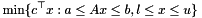where for some given old dimensions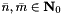the data are(linear cost,lower and upper bounds on the variables),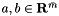(lower and upper bounds on the constraint values),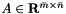(the constraint matrix). In addition the class supports manipulating a vector of starting values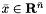. These not be feasible for the data (for some infeasible methods this might even be desirable), so their values are not checked. If desired, checking feasibility with respect to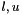can be turned on.

Supported are an arbitrary sequence of

• setting new values for lower/upper bounds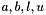on selected indices (add_set_lb(), add_set_ub(), add_set_rhslb(), add_set_rhsub()), by the default consistency of the bounds is checked when applying the changes (typically it cannot be done before in lack of the necessary information regarding the opposite bound or would require simultaneous setting of lower and upper bounds which most applications do not want to do).
• appending variables (add_append_vars()), i.e. appending further elements to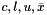as well as further columns to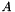.
• reassigning the variables (add_reassign_vars()) via an index vector whose entries specify which variable will be moved to this position. Each existing variable index may appear at most once in this index vector, but not all indices need to appear. If they do not appear they will be deleted. This affectsand the columns of.
• deleting variables (add_delete_vars()); the indices to be deleted are specfied by an index vector, which is then used to generates an index vector as a map to the old indices and then uses this with the previous reassign variables.
• appending constraints rows (add_append_rows()), i.e. appending further rows toand further elements to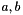.
• reassigning the constraint rows (add_reassign_rows()) in the same style as reassigning variables
• deleting constraint rows (add_delete_rows()) in the same style as deleting variables

Each next modification has to be given/added with respect to the current virtual number of variables and constraint rows as if the compiled modifications had been carried out already. If certain elements are not specified, they are assumed to have the following default values (if used at all; they can also be set to other values on construction or by clear()):

• 0 for the linear cost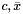and matrix elements of• CB_minus_infinity for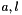• CB_plus_infinity for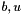The modifications are compiled within this class (and not yet executed) to one bulk of data of bound inromations, appending information for variables and rows and reassignment information, so that the entire sequence of transformations is then achieved in the following sequence of four steps (the sequence is important here!)

1. setting the requested bounds to their new values
2. appending the compiled variable (and column) data
3. reassigning the variables (and columns) by the compiled variable reassignment map
4. appending the compiled constraint row data
5. reassigning the constraint rows by the compiled row reassignment map

Another modification can be incorporated into this one if either its starting dimensions match the current values or if the modification starts from the same old dimensions and only appends information. In the latter case any "missed" appensions of *this are executed with the neutral element, likewise the reassignments of *this are executed and then the resultig modified parts of the modification to incorporate are appended.

In the end the routines apply_to_vars() and apply_to_rows() can be used to carry out the modifications.

Member Function Documentation

 int ConicBundle::Modification::add_append_rows ( CH_Matrix_Classes::Integer append_dim, const CH_Matrix_Classes::Sparsemat * append_rows, const CH_Matrix_Classes::Matrix * append_rhslb, const CH_Matrix_Classes::Matrix * append_rhsub )

append information on new rows at the respective ends

Parameters
 append_dim number of rows (constraints) to be appended append_rows if NULL, append default values, otherwise it must point to a sparse matrix of size append_dim times new_vardim() that is to be appended to the constraint matrix below. append_rhslb if NULL, append default values, otherwise it must point to a column vector of size append_dim that is to be appended to the vector of right hand side lower bounds append_rhsub if NULL, append default values, otherwise it must point to a column vector of size append_dim that is to be appended to the vector of right hand side upper bounds
Returns
number of errors; if errors occured, none of the new changes are performed

 int ConicBundle::Modification::add_append_vars ( CH_Matrix_Classes::Integer append_dim, const CH_Matrix_Classes::Matrix * append_lb, const CH_Matrix_Classes::Matrix * append_ub, const CH_Matrix_Classes::Sparsemat * append_cols, const CH_Matrix_Classes::Matrix * append_start_val, const CH_Matrix_Classes::Matrix * append_linear_costs )

append information on new variables at the respective ends

Parameters
 append_dim number of variables (or columns of the constraint matrix) to be appended append_lb if NULL, append default values, otherwise it must point to a column vector of size append_dim that is to be appended to the vector of lower bounds on the variable values. Errors occur whenever a value is greater than CB_plus_infinity or the corresponding upper bound (if specified). Warnings are issued whenever a value equals CB_plus_infinity, is smaller than CB_minus_infinity or is greater than the corresponding start_val (if given). append_ub if NULL, append default values, otherwise it must point to a column vector of size append_dim that is to be appended to the vector of upper bounds on the variable values Errors occur whenever a value is smaller than CB_minus_infinity or the corresponding lower bound (if specified). Warnings are issued whenever a value equals CB_minus_infinity, is greater than CB_plus_infinity or is smaller than the corresponding start_val (if given). append_cols if NULL, append default values, otherwise it must point to a sparse matrix of size new_rowdim() times append_dim that is to be appended to the constraint matrix on the right append_start_val if NULL, append default values, otherwise it must point to a column vector of size append_dim that is to be appended to the vector of starting values for the variables. Starting values may violate the bounds, but this causes a warning. append_linear_costs if NULL, append default values, otherwise it must point to a column vector of size append_dim that is to be appended to the cost vector for the variables
Returns
number of errors; if errors occured, none of the new changes are performed

 int ConicBundle::Modification::add_delete_rows ( const CH_Matrix_Classes::Indexmatrix & del_ind, CH_Matrix_Classes::Indexmatrix & map_to_old )

delete the rows indexed by the vector del_ind and return the index changes of the others in a vector map_to_old

del_ind need not be ordered in any way, but any index in 0 to new_rowdim()-1 may appear at most once. On output the dimension of the column vector map_to_old gives the number of remaining rows and its entry i holds the index the row with new index i had before the deletion. The return value is the number of errors in del_ind. If such occured, this deletion is not performed and map_to_old may contain garbage.

 int ConicBundle::Modification::add_delete_vars ( const CH_Matrix_Classes::Indexmatrix & del_ind, CH_Matrix_Classes::Indexmatrix & map_to_old )

delete the variables indexed by the vector del_ind and return the index changes of the others in a vector map_to_old

del_ind need not be ordered in any way, but any index in 0 to new_vardim()-1 may appear at most once. On output the dimension of the column vector map_to_old gives the number of remaining variables and its entry i holds the index the variable with new index i had before the deletion. The return value is the number of errors in del_ind. If such occured, this deletion is not performed and map_to_old may contain garbage.

 int ConicBundle::Modification::add_reassign_rows ( const CH_Matrix_Classes::Indexmatrix & map_to_old )

reassign the current row indices (with modifications) as specified by map_to_old

map_to_old must specify an injective map (no two values match) into indices 0 up to new_rowdim()-1 (not all need to appear). The row getting index i (for i=0 to map_to_old.dim()-1) is the row with current index map_to_old(i) (current refers to considering all previous modifications as having been carried out already). The return value is the number of errors in map_to_old. If such occured, this reassign is not performed.

 int ConicBundle::Modification::add_reassign_vars ( const CH_Matrix_Classes::Indexmatrix & map_to_old )

reassign the current variable indices (with modifications) as specified by map_to_old

map_to_old must specify an injective map (no two values match) into indices 0 up to new_vardim()-1 (not all need to appear). The variable getting index i (for i=0 to map_to_old.dim()-1) is the variable with current index map_to_old(i) (current refers to considering all previous modifications as having been carried out already). The return value is the number of errors in map_to_old. If such occured, this reassign is not performed.

 int ConicBundle::Modification::add_set_lb ( CH_Matrix_Classes::Integer ind, CH_Matrix_Classes::Real lb )

set the lower bound on variable with index ind to value lb

If value lb exceeds CB_plus_infinity or the index is out of range, the return value is 1 and no changes are carried out, otherwise it returns 0.

 int ConicBundle::Modification::add_set_rhslb ( CH_Matrix_Classes::Integer ind, CH_Matrix_Classes::Real rhslb )

set the lower bound on row right hand side with index ind to value rhslb

If value rhslb exceeds CB_plus_infinity or the index is out of range, the return value is 1 and no changes are carried out, otherwise it returns 0.

 int ConicBundle::Modification::add_set_rhsub ( CH_Matrix_Classes::Integer ind, CH_Matrix_Classes::Real rhsub )

set the upper bound on row right hand side with index ind to value rhsub

If value rhsub is below CB_minus_infinity or the index is out of range, the return value is 1 and no changes are carried out, otherwise it returns 0.

 int ConicBundle::Modification::add_set_ub ( CH_Matrix_Classes::Integer ind, CH_Matrix_Classes::Real ub )

set the upper bound on variable with index ind to value ub

If value ub is below CB_minus_infinity or the index is out of range, the return value is 1 and no changes are carried out, otherwise it returns 0.

◆ apply_to_rows()

 int ConicBundle::Modification::apply_to_rows ( CH_Matrix_Classes::Sparsemat * rows, CH_Matrix_Classes::Matrix * rhslb, CH_Matrix_Classes::Matrix * rhsub ) const

carry out the collected modifications on the given vectors

If a specific parameter is NULL, no changes are performed on it. If it is not null, rows must point to a sparse matrix of size old_rowdim() times old_vardim(), rhslb or rhsub must point to a column vector of length old_rowdim(). Then the following operations will be performed on it in this sequence (variable/column operations only apply to rows) :

1. new column information is appended to rows (or default values, if the corresponding append information pointer is NULL)
2. if column reassignment information is given, the columns of rows are mapped as given by *map_to_old_variables()
3. new row information is appended (or default values, if the corresponding append information pointer is NULL)
4. if row reassignment information is given, the rows are mapped as given by *map_to_old_rows()
Parameters
 [in,out] rows if not NULL, this points to the old sparse matrix of constraint rows. [in,out] rhslb if not NULL, this points to the old vector of right hand side lower bounds [in,out] rhsub if not NULL, this points to the old vector of right hand side upper bounds
Returns
the number of dimension errors of non NULL inputs, if any, no modifications are made to any inputs.

Referenced by ConicBundle::LPGroundsetModification::apply_to_rows().

◆ apply_to_vars()

 int ConicBundle::Modification::apply_to_vars ( CH_Matrix_Classes::Matrix * vars, CH_Matrix_Classes::Matrix * lb, CH_Matrix_Classes::Matrix * ub, CH_Matrix_Classes::Matrix * cost ) const

carry out the collected modifications on the given vectors

If a specific parameter is NULL, no changes are performed on it, if it is not null, it must point to a column vector of length old_vardim(). Then the following operations will be performed on it in this sequence:

1. new information is appended (or default values, if the corresponding append information pointer is NULL)
2. if reassignment information is given, the values are mapped as given by *map_to_old_variables()
Parameters
 [in,out] vars if not NULL, this points to the old vector of variable values. The start values will be appended to it before a possible index reassignment. [in,out] lb if not NULL, this points to the old vector of lower bounds on the variables. [in,out] ub if not NULL, this points to the old vector of upper bounds on the variables [in,out] cost if not NULL, this points to the old cost vector
Returns
the number of dimension errors of non NULL inputs, if any, no modifications are made to any inputs.

◆ clear()

 int ConicBundle::Modification::clear ( CH_Matrix_Classes::Integer var_olddim, CH_Matrix_Classes::Integer row_olddim, bool ensure_start_val_box_feasibility = false, bool ensure_bounds_consistency = true, CH_Matrix_Classes::Real start_val_def = 0., CH_Matrix_Classes::Real bounds_minus_infty = CB_minus_infinity, CH_Matrix_Classes::Real bounds_plus_infty = CB_plus_infinity, CH_Matrix_Classes::Real rhs_minus_infty = CB_minus_infinity, CH_Matrix_Classes::Real rhs_plus_infty = CB_plus_infinity, CH_Matrix_Classes::Real cost_def = 0. )

resets all variables so that the object to be modified has starting size var_olddim (number of variables) and row_olddim (number of rows) and no modifications

The actual old data is not needed at this point, the changes on it will be collected and excuted in the routines apply_to_vars and apply_to_rows

Setting the parameter ensure_start_val_box_feasibility to true will cause the algorithm to check in add_append_vars() whether the input values are within the given bounds and in apply_to_vars() it will project all start_values onto the bounds for all, old and new, indices (which might have been changed by then). If it is false (default), all values will be accepted as given.

Setting the parameter ensure_bounds_consistency to true (default) will raise errors in add_append_vars() and in apply_to_vars() whenever there are lower bounds greater than the respective upper bounds so as to avoid trivial infeasibilities. This check is omitted if set to false.

The remaining values give the values of plus and minus infinity the no bounds should exceed. These are the default values at the same time (maybe it might be good to have a separate default value, but this is not implemented here).

◆ incorporate()

 virtual int ConicBundle::Modification::incorporate ( const Modification & m )
virtual

add the modification specified in m on top of the modifications collected so far

For this, the old_vardim() of modification m must be identical to new_vardim() of this and old_rowdim() of modification m must be identical to new_rodim() of this. The return value is the number of errors in this respect. If such occured, this incorporation is not performed.

The documentation for this class was generated from the following file: